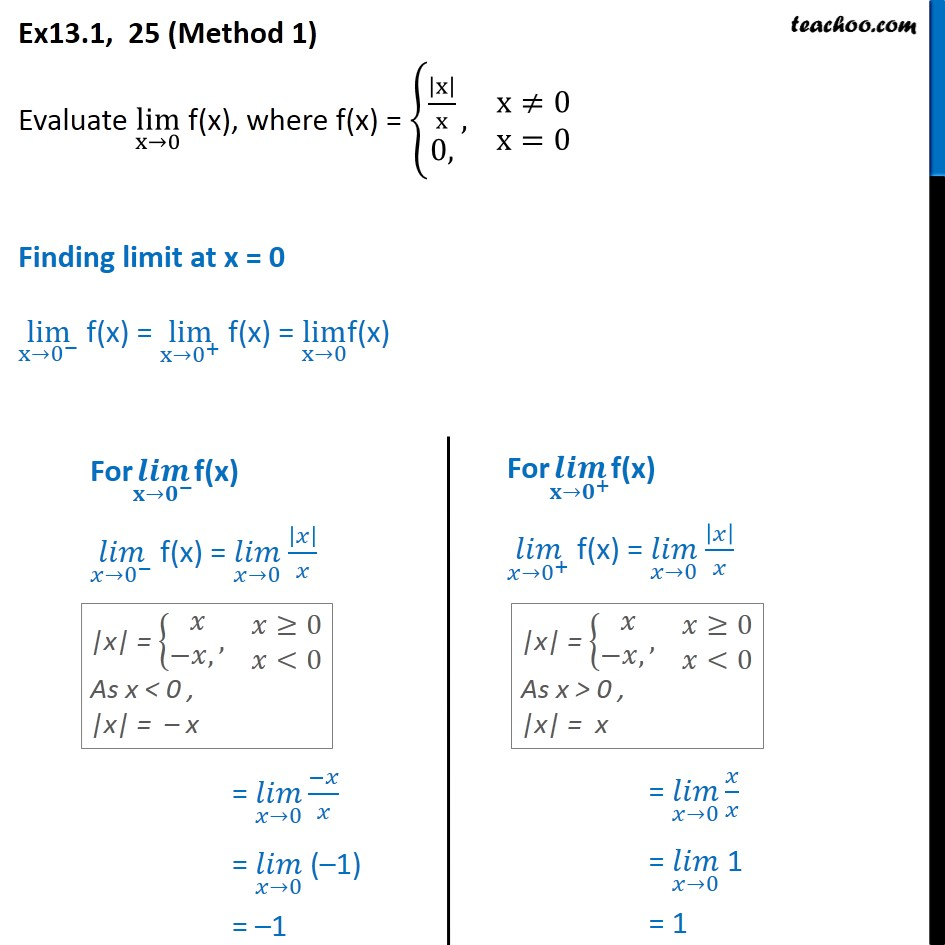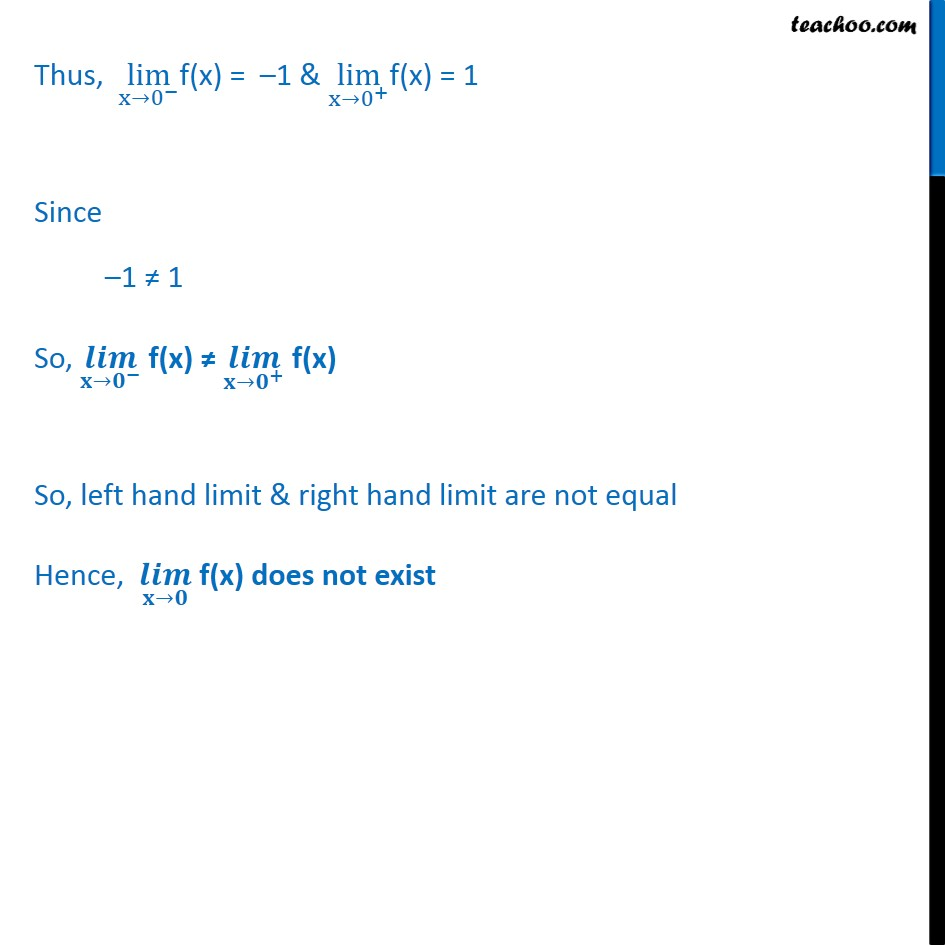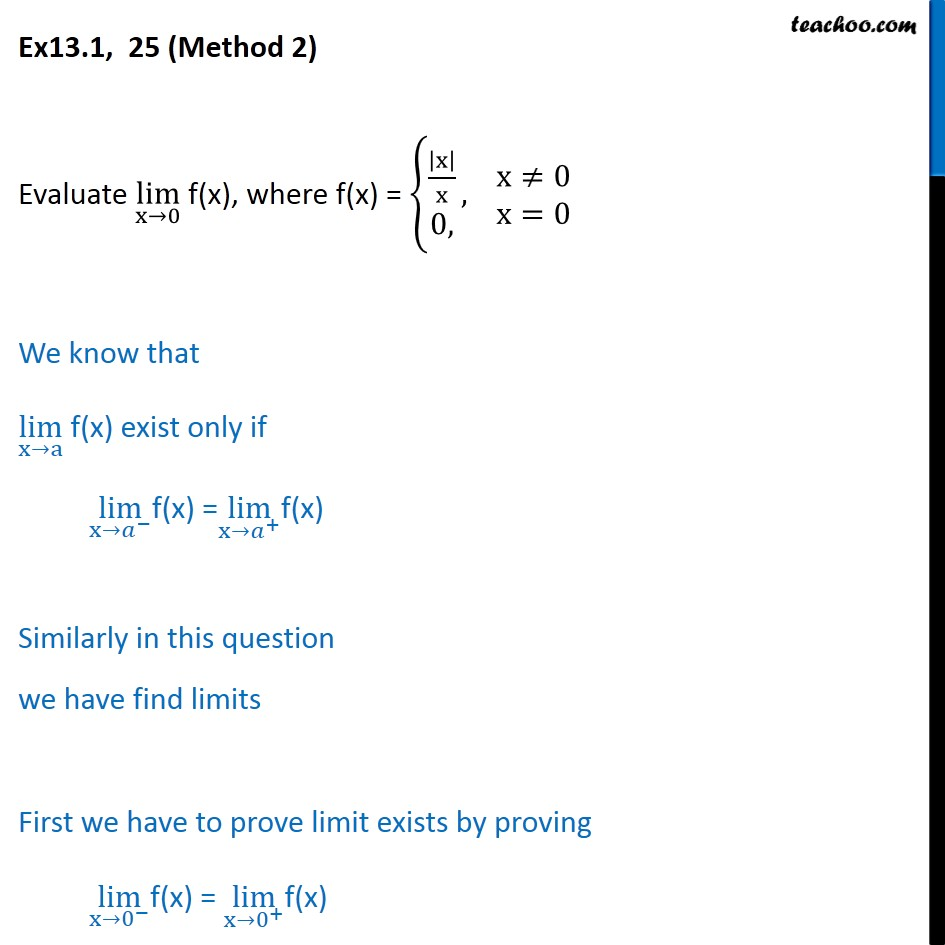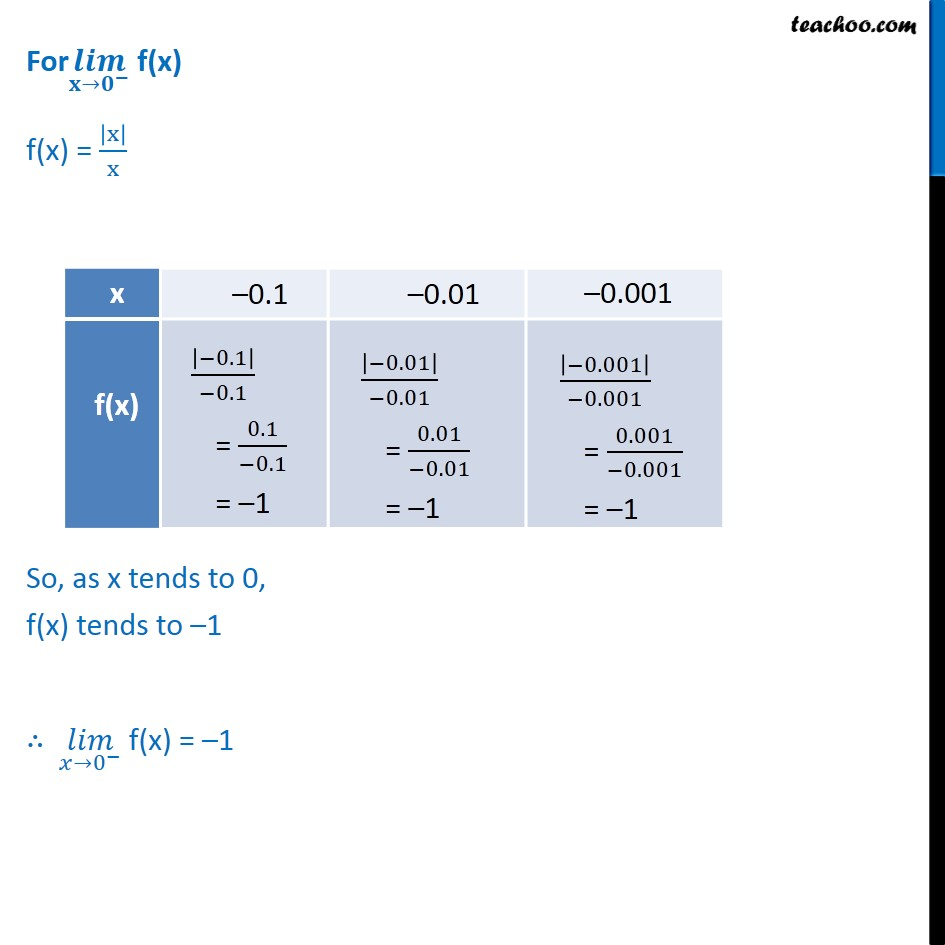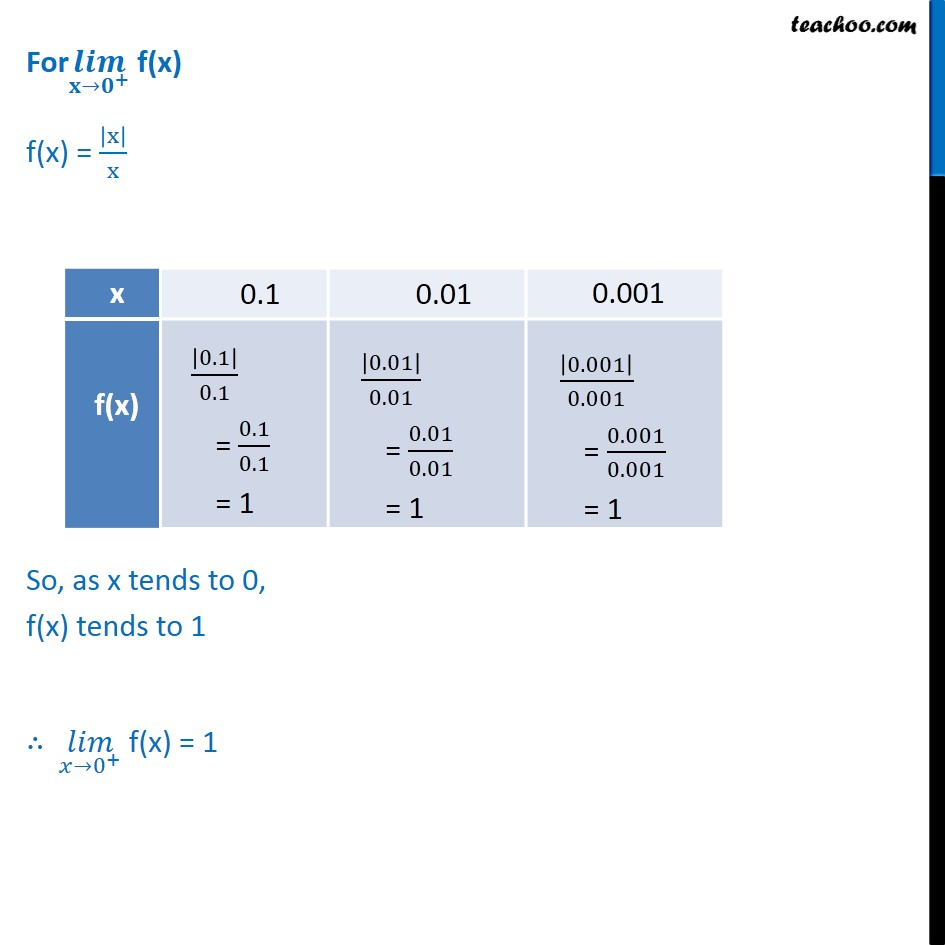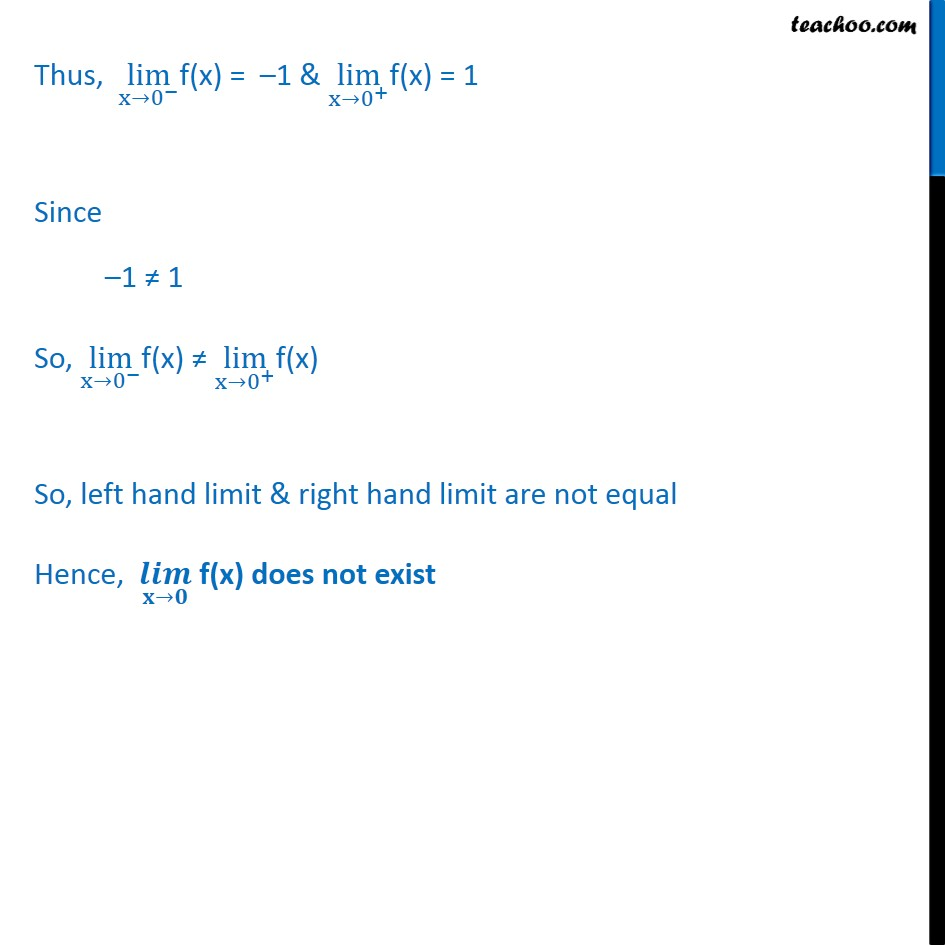1. Chapter 13 Class 11 Limits and Derivatives
2. Serial order wise
3. Ex 13.1

Transcript

Ex 13.1, 25 (Method 1) Evaluate lim x 0 f(x), where f(x) = x x 0, , x 0 x=0 Finding limit at x = 0 lim x 0 f(x) = lim x 0 + f(x) = lim x 0 f(x) Thus, lim x 0 f(x) = 1 & lim x 0 + f(x) = 1 Since 1 1 So, f(x) + f(x) So, left hand limit & right hand limit are not equal Hence, f(x) does not exist Ex13.1, 25 (Method 2) Evaluate lim x 0 f(x), where f(x) = x x 0, , x 0 x=0 We know that lim x a f(x) exist only if lim x f(x) = lim x + f(x) Similarly in this question we have find limits First we have to prove limit exists by proving lim x 0 f(x) = lim x 0 + f(x) For f(x) f(x) = x x So, as x tends to 0, f(x) tends to 1 0 f(x) = 1 For + f(x) f(x) = x x So, as x tends to 0, f(x) tends to 1 0 + f(x) = 1 Thus, lim x 0 f(x) = 1 & lim x 0 + f(x) = 1 Since 1 1 So, lim x 0 f(x) lim x 0 + f(x) So, left hand limit & right hand limit are not equal Hence, f(x) does not exist

Ex 13.1